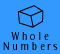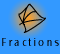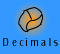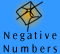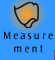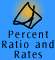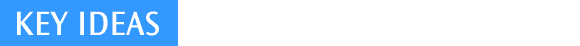|Meaning of operations| Associative and Commutative Laws |Inverse operations |
Distributive Law | Order of Operations | Estimation and Mental Computation | Quick quiz |

Meaning of operations

There are four basic operations in arithmetic: addition, subtraction, multiplication and division. It is important to understand the types of problems which each of these solves. Meaning is constructed through experience with materials and real life situations and through the language used to discuss what is happening. Only when an understanding of at least an initial meaning for an operation has been developed, and simple mental calculations can be carried out, should formal algorithms be introduced. A range of meanings for the operations is needed to illustrate arithmetic algorithms thoroughly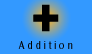There are two basic meanings for addition. Firstly, there is the notion of combining sets, where two separate sets are joined together. For example, if there are 16 boys in the class and 10 girls, there are 26 children altogether. Secondly, there is the notion of making one set larger. For example, if there are 16 boys in the class and another one comes, there are now 17 boys. Children learn both of these meanings for addition early.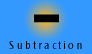There are two basic meanings for subtraction. Firstly, there is the notion of take away. For example, if I have 16 boys in the class and two leave, there are only 14 left. There is also the notion of comparison (e.g. what is the difference in age between a child who is 7 and one who is 9?). Take away is the easiest form and is introduced first. Meaningful language such as take away and difference between will be used before the introduction of subtract.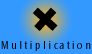Multiplication begins with the notion of equal groups (e.g. 3 groups of 4 lollies makes 12 lollies altogether). This form is expanded to arrays so students see equal groups represented in rows and columns. Later, it is further generalised to rates (e.g. speed = distance x time). Also beginning in the early years, is the notion of multiplicative comparison (e.g. my house is three quarters as big as yours). The language phrases for multiplicative comparison include 'three times as many as', 'three times as big as' and 'half as tall as' and use of percentages.The division operation is most easily explained using the shared between notion, called partition division. This approach is easily modelled with concrete materials. Division can also be viewed as asking how many groups there are of a specified size, called quotition division. For example, 6÷2 can be explained as either 'if 6 things are shared amongst 2 people, how many does each person get?' ( 3 things each, partition) or 'how many groups of 2 things can be made from 6 things'? (3 groups, quotition). Later, there are also other meanings.

The table above describes the most common meanings for each of the operations. Note, however, that addition situations will give rise to problems to be solved by addition or by subtraction and that subtraction situations give rise to problems which are solved by addition. For example, consider the situation where I have some lollies and then I eat 3. This is a take away situation. However, if I know that there are 6 left, I can find out how many I had first by adding, not by subtracting. The same cross-over holds for multiplication and division.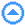Associative and Commutative Laws

By appreciating the meaning of the operations, we can deduce their basic properties and then the basic facts. Knowledge of the basic facts of the four operations and place value is then the foundation for the algorithms. There are many basic properties which follow from two important principles: the commutative and associative laws for addition and multiplication.

a + b = b + a for all real numbers a and b

Reason: when two sets are combined, the total number of objects does not depend on which of the sets was placed down first.

(a + b) + c = a + (b + c) for all real numbers a, b and c

Reason: when three sets are combined, the total number of objects does not depend on whether you combine the first two sets and then add the third, or start with the first and add the second and third combined.

Commutative law for multiplication

a x b = b x a for all real numbers a and b

Reason: This can best be shown using arrays. It is explained in the Teaching Connections section.

Associative law for multiplication

(a x b) x c = a x (b x c) for all real numbers a, b and c

Reason: This can best be shown using a three dimensional array. The number of objects in the array does not depend on whether the number in a horizontal cross-section is calculated first and multiplied by the number of horizontal layers, or if the number of vertical slices is multiplied by the number of objects in a vertical cross-section.

Uses of the laws for addition

The commutative law means we only have to learn half the basic addition facts because we know that order does not matter for adding, e.g. 8 + 4 = 4 + 8. The associative law allows us to group numbers in convenient ways to add them, e.g. to add 8 and 5, we can split the 5 into 2 + 3 and use the known combination of 2 + 8 : 8 + 5 = 8 + (2 + 3) = (2 + 8) + 3 = 10 + 3 = 13.

In the example below we have used these laws to reorder and regroup numbers to quickly add 38 and 14. The steps written out seem long, but they are carried out very fast.

Example 1: 38 + 14 = 52

 38 + 14 = 38 + (12 + 2) = 38 + (2 + 12) (commutative law) = (38 + 2) + 12 (associative law) = 40 + 12 = 52

Uses of the laws for multiplication

The commutative law means we only have to learn half the basic multiplication facts because we know that order does not matter , e.g. 8 x 4 = 4 x 8. The associative law allows us to group numbers in convenient ways to multiply them, e.g. to multiply 5 and 16, we can split the 16 into 8 x 2 and use the known combination of 5 x 8 and the easy calculation of 40 x 2: 5 x 16 = 5 x (8 x 2) = (5 x 8) x 2 = 40 x 2 = 80.

In the example below we have used these laws to reorder and regroup numbers to quickly multiply 75 by 4. The steps written out seem long, but they are carried out very fast.

Example 2: 75 x 4 = 300

 75 x 4 = (25 x 3) x 4 = (3 x 25) x 4 (commutative law) = 3 x (25 x 4) ( associative law) = 3 x 100 = 300

Inverse operations

Subtraction is the inverse operation of addition and division is the inverse operation of multiplication.

Because it is the inverse operation, subtracting a number "undoes" adding it

e.g. 8 + 27 -27 = 8 and 8 - 27 + 27 = 8.

Because it is the inverse operation, dividing by a number "undoes" multiplying by it

e.g. 8 x 27 ÷ 27 = 8 and 8 ÷ 27 x 27 = 8.

The properties of subtraction and division (which are NOT commutative nor associative) follow from their inverse status.

Children learn basic subtraction facts alongside basic addition facts and basic division facts alongside multiplication facts.

Also note that addition is the inverse operation of subtraction and multiplication is the inverse operation for division.Distributive lawOrder of operations

The order of operations is fundamental to working with numbers. A comprehensive explanation of the correct order of operations, including common misconceptions and appropriate rules, can be found by clicking here, Order of Operations.

Estimation and mental computation

The skills of estimation and mental computation are built on the understanding of the basic concepts for each operation and use knowledge of basic facts, place value and rounding. Estimation and mental computation are needed to provide both approximate answers in many real life situations and to check reasonableness of written and calculator answers.

Rounding numbers so that they are easy to deal with is a key skill for quick estimates.

Example: How can I round the following numbers to the nearest ten?

 53 53 has 5 tens, but there are only 3 ones so it is closer to 50 than 60 97 97 has 9 tens and 7 ones. 7 ones is closer to 10 ones than no ones, so I round the number up to 100 65 65 has 6 tens and 5 ones. 5 ones is not closer to 0 than 10, it is in the middle. I could round this number up or down but rounding up to 70 is more usual.

In calculations, it is important to appreciate the effect of rounding up or down. For example, when I estimate 29 x 37 by 30 x 40 = 1200, the answer is too big. If I estimate 29 divided by 37 by 30 divided by 40 = 0.75, I do not immediately know whether that answer is too big or too small.Quick quiz

| Set A| Set B | Set C | Set D |

Set A:

 1. 2 x 87 x 5 = 10 x ? 2. 5 x 16 x 4 = 20 x ? 3. 5 x 16 x 4 = 80 x ? 4. 2 x 10 x 40 = 2 x ? 5. 2 x 10 x 40 = 80 x ? 6. 25 x 5 x 4 = 100 x ? 7. 6 x 12 x 5 = 12 x ? 8. 2 x 8 x 7 = 14 x ? 9. 9 x 3 x 10 = 90 x ? 10. 250 x 6 x 4 = 1000 x ?

Set B:

 1 27+23 = 27 + 3 + ? 2 16+46 = 46 + 14 + ? 3 18 + 33 = 33 + 17 + ? 4 75 + 28 = 75 + 25 + ? 5 113 + 48 = 113 + 47 + ? 6 87 + 14 = 87 + 13 + ? 7 1094 + 9 = 1094 + 6 + ? 8 449 + 23 = 449 + 21 + ? 9 1003 + 1103 = 1000 + 1000 + ? 10 1003 + 1103 = 1000 + 1000 + ?

Set C:

 1 18 + 6 - 6 =? 2 324 + 156 - 324 =? 3 98 x 29 ÷ 29 =? 4 184 x 236 ÷ 184 =? 5 256 x 943 ÷ 256 + 149 - 149 =?

Set D:

 Write the following as a number sentence in as many ways as possible using only the given numbers and solve: 1. Annie has 75 jazz CDs and 135 pop CDs. How many CDs does she have altogether? 2. At a party there are 19 girls and 27 boys. When 13 more girls arrive, how many people are at the party? 3. In a restaurant there are 4 people at each of 23 tables. How many people are there? 4. Abdul writes a 2 page essay. He types 25 words to a line and 50 lines to a page. How many words does he write in his essay? 5. At the park there are 16 dogs each with 4 legs and 12 owners each with 2 legs. How many legs are there? 6. In a class room there are 14 tables and 84 chairs. How many legs are there on all the tables and chairs combined if each piece of furniture has four legs? 7. Five girls have three dresses each with four buttons per dress and seven girls have five dresses each with 2 buttons per dress. How many buttons are there? 8. A farmer wants to approximate the number of pieces of fruit on the trees in his orchard. In the orchard there are 120 lemon trees each with about 80 lemons on them and 230 orange trees each with about 60 oranges on them. Approximately, how many pieces of fruit are there? 9. Twenty girls have four CDs each and each CD has 16 songs on it. How many songs are there altogether? 10. In a particular street there are 20 houses. 9 of the houses receive 3 newspapers each day and the remainder of the houses get 2 newspapers daily. How many newspapers are purchased by the occupants of these houses each day?